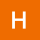Home
IT Knowledge
Inspiration
Languages
EN

# Java 8 - stream average

0 points
Created by:halfera
291

In this article, we would like to show you how to find stream average in Java 8.

Quick solution:

``````// example stream
Stream<Integer> streamOfIntegers = Stream.of(1, 2, 3);

streamOfIntegers
.mapToDouble(x -> x)
.average()
.ifPresent(avg -> System.out.println("average = " + avg));  // 2.0``````

## Practical example

In this example, we use:

• `mapToInt()` - to map each element of the stream to int,
• `average()` - to calculate the average element of the stream,
• `ifPresent()` - to display the average if the value is present.
``````import java.util.stream.Stream;

public class Example {

public static void main(String[] args) {
// example stream
Stream<Integer> streamOfIntegers = Stream.of(1, 2, 3);

// find stream average
streamOfIntegers
.mapToInt(x -> x)
.average()
.ifPresent(avg -> System.out.println("average = " + avg));
}
}``````

Output:

``average = 2.0``